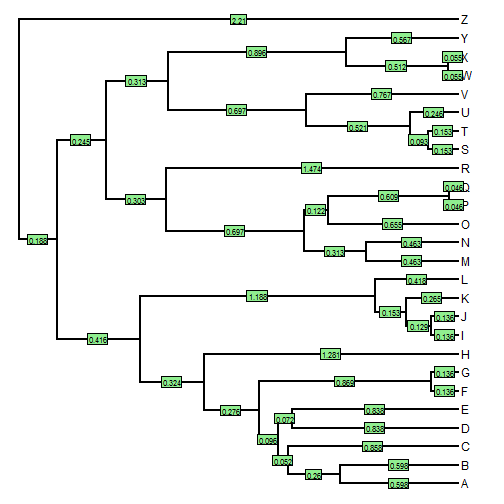## Tuesday, February 23, 2016

### Extracting the terminal edge lengths for a set of tips

A phytools user asked the following:

Is there a good way to extract the branch length for a given set of tips? For a given tree, we would like to calculate the mean and variance for the terminal branch lengths leading to a specific set of tips. Is there a way in phytools to target a set of tips and extract those branch lengths?

If I understand the question properly, then what we have is a tree:

``````tree
``````
``````##
## Phylogenetic tree with 26 tips and 25 internal nodes.
##
## Tip labels:
##  A, B, C, D, E, F, ...
##
## Rooted; includes branch lengths.
``````

and a set of tips:

``````tips
``````
``````##   "K" "V" "P" "S" "J" "Q" "X" "B" "H" "D"
``````

and we want to extract the set of terminal edge lengths associated with these tips. This is easy.

``````## first get the node numbers of the tips
nodes<-sapply(tips,function(x,y) which(y==x),y=tree\$tip.label)
## then get the edge lengths for those nodes
edge.lengths<-setNames(tree\$edge.length[sapply(nodes,
function(x,y) which(y==x),y=tree\$edge[,2])],names(nodes))
``````

We can check as follows:

``````## our edge lengths
edge.lengths
``````
``````##          K          V          P          S          J          Q
## 0.26489330 0.76661579 0.04553788 0.15279683 0.13592584 0.04553788
##          X          B          H          D
## 0.05511474 0.59774131 1.28135176 0.83764106
``````
``````plotTree(tree)
edgelabels(round(tree\$edge.length,3),cex=0.7)
``````That's all there is to it.

The data used for this exercise were simulated as follows:

``````library(phytools)
tree<-pbtree(n=26,tip.label=LETTERS)
tips<-sample(LETTERS,10)
``````

1.Hi Liam,

How would it change in case of a multiphylo object?

Cheers

2.The Bestreplica watches. Here you can find almost swiss brand replica watches.Replica watches,one of the most famous brands,best rolex ,Specialities watch for sale,Fast delivery and free shipping!

Note: due to the very large amount of spam, all comments are now automatically submitted for moderation.